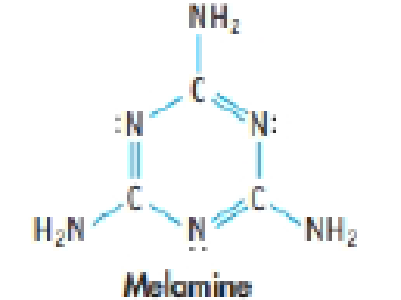Chapter 9, Problem 70SCQ

Chapter
Section
Textbook Problem

Melamine is an important industrial chemical, used to make fertilizers and plastics.(a) The carbon-nitrogen bond lengths in the ring are all the same length (about 140 pm). Explain.(b) Melamine is made by the decomposition of urea, (H2N)2CO.6 (H2N)2CO(s) → C3H6N6(s) + 6 NH3(g) + 3 CO2(g)Calculate the enthalpy change for this reaction. Is it endo- or exothermic? [ΔfH° for melamine(s) = –66.1 kJ/mol and for urea(s) = –333.1 kJ/mol]

(a)

Interpretation Introduction

Interpretation:

The explanation for the same carbon-nitrogen bond length in the melamine ring has to be given.

Concept Introduction

Bond length: It is defined as the distance between the centers of two covalent bonded atoms. The higher bond order the stronger the pull between the two atoms and the shorter the bond length.

Hybridization is the mixing of valence atomic orbitals to get equivalent hybridized orbitals that having similar characteristics and energy.

• Geometry of a molecule can be predicted by knowing its hybridization.
• sp3  hybrid orbitals is produced by hybridization of single s-orbital and three p-orbital.
• sp2  hybrid orbitals is produced by hybridization between one s-orbital and two p-orbitals.
• sp  hybrid orbitals is produced by hybridization of single s-orbital and single p-orbital.
Explanation

The bond length of CN bond in the melamine molecule is same.

(b)

Interpretation Introduction

Interpretation:

The enthalpy change for the production of melamine by the decomposition of urea has to be given.

Concept Introduction

Enthalpy is the amount of energy absorbed or released in a process.

The enthalpy change in a system Ηsys) can be calculated by the following equation.

ΔH°=nHfproductsοmHfReactantsο

Where,

ΔH°reactants is the standard enthalpy of the reactants

ΔH°produdcts is the standard enthalpy of the products

Still sussing out bartleby?

Check out a sample textbook solution.

See a sample solution

The Solution to Your Study Problems

Bartleby provides explanations to thousands of textbook problems written by our experts, many with advanced degrees!

Get Started